# How to Calculate and Solve for Standing Bubble Point | The Calculator Encyclopedia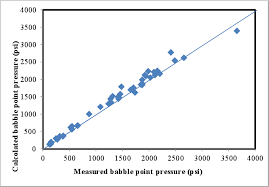The image above represents standing bubble point.

To compute for the standing bubble point, three essential parameters are needed and these parameters are Specific Gravity of Solution Gas (γg), Bubble Point Pressure (Pb) and Standing Bubble Point Parameter (a).

The formula for calculating the standing bubble point:

Rs = 18.2[((Pb / γg)0.83 x 10a) – 1.4]

Where:

Rs = Standing Bubble Point
γg = Specific Gravity of Solution Gas
Pb = Bubble Point Pressure
a = Standing Bubble Point Parameter

Let’s solve an example;
Find the standing bubble point with a specific gravity of solution gas of 24, bubble point pressure of 70 and standing bubble point parameter of 45.

This implies that;

γg = Specific Gravity of Solution Gas = 24
Pb = Bubble Point Pressure = 70
a = Standing Bubble Point Parameter = 45

Rs = 18.2[((Pb / γg)0.83 x 10a) – 1.4]
Rs = 18.2[((70 / 24)0.83 x 1045) – 1.4]
Rs = 18.2[((2.916)0.83 x 1045) – 1.4]
Rs = 18.2[(2.43 x 1045) – 1.4]
Rs = 18.2[2.431e+45 – 1.4]
Rs = 18.2[2.431e+45]
Rs = 4.425e+46

Therefore, the standing bubble point is 4.425e+46.

Nickzom Calculator – The Calculator Encyclopedia is capable of calculating the standing bubble point.

To get the answer and workings of the standing bubble point using the Nickzom Calculator – The Calculator Encyclopedia. First, you need to obtain the app.

You can get this app via any of these means:

To get access to the professional version via web, you need to register and subscribe for NGN 1,500 per annum to have utter access to all functionalities.
You can also try the demo version via https://www.nickzom.org/calculator

Apple (Paid) – https://itunes.apple.com/us/app/nickzom-calculator/id1331162702?mt=8
Once, you have obtained the calculator encyclopedia app, proceed to the Calculator Map, then click on Petroleum under EngineeringNow, Click on Fluid Properties under Petroleum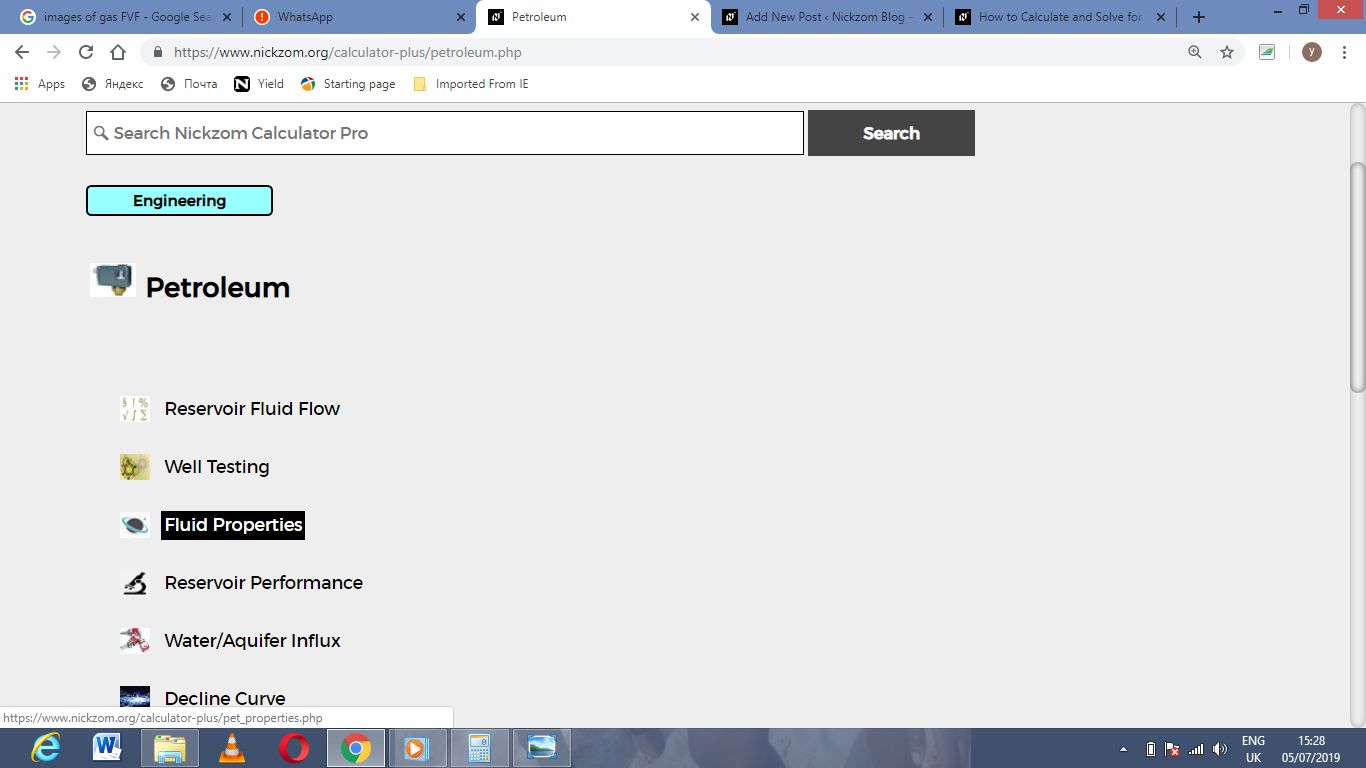Now, Click on Standing Bubble Point under Fluid PropertiesThe screenshot below displays the page or activity to enter your values, to get the answer for the standing bubble point according to the respective parameters which are the Specific Gravity of Solution Gas (γg), Bubble Point Pressure (Pb) and Standing Bubble Point Parameter (a).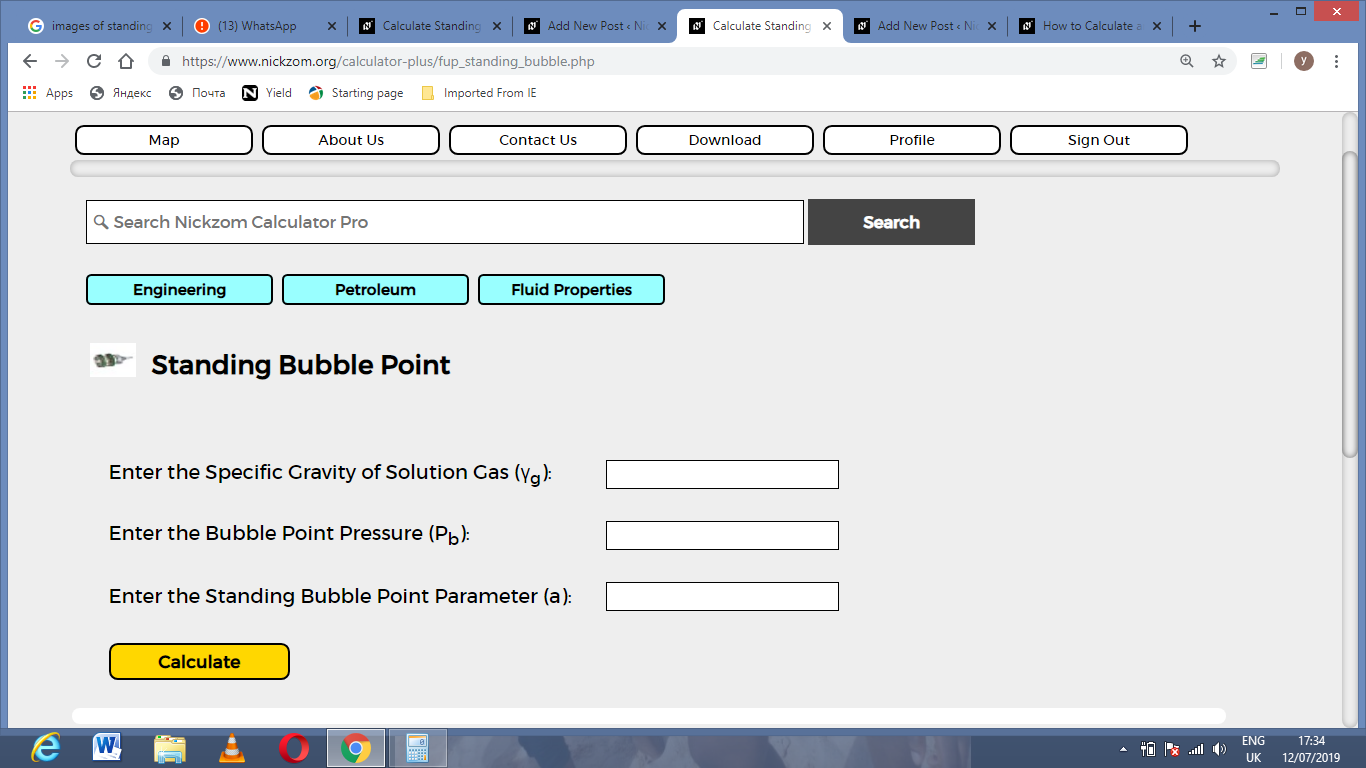Now, enter the values appropriately and accordingly for the parameters as required by the Specific Gravity of Solution Gas (γg) is 24, Bubble Point Pressure (Pb) is 70 and Standing Bubble Point Parameter (a) is 45.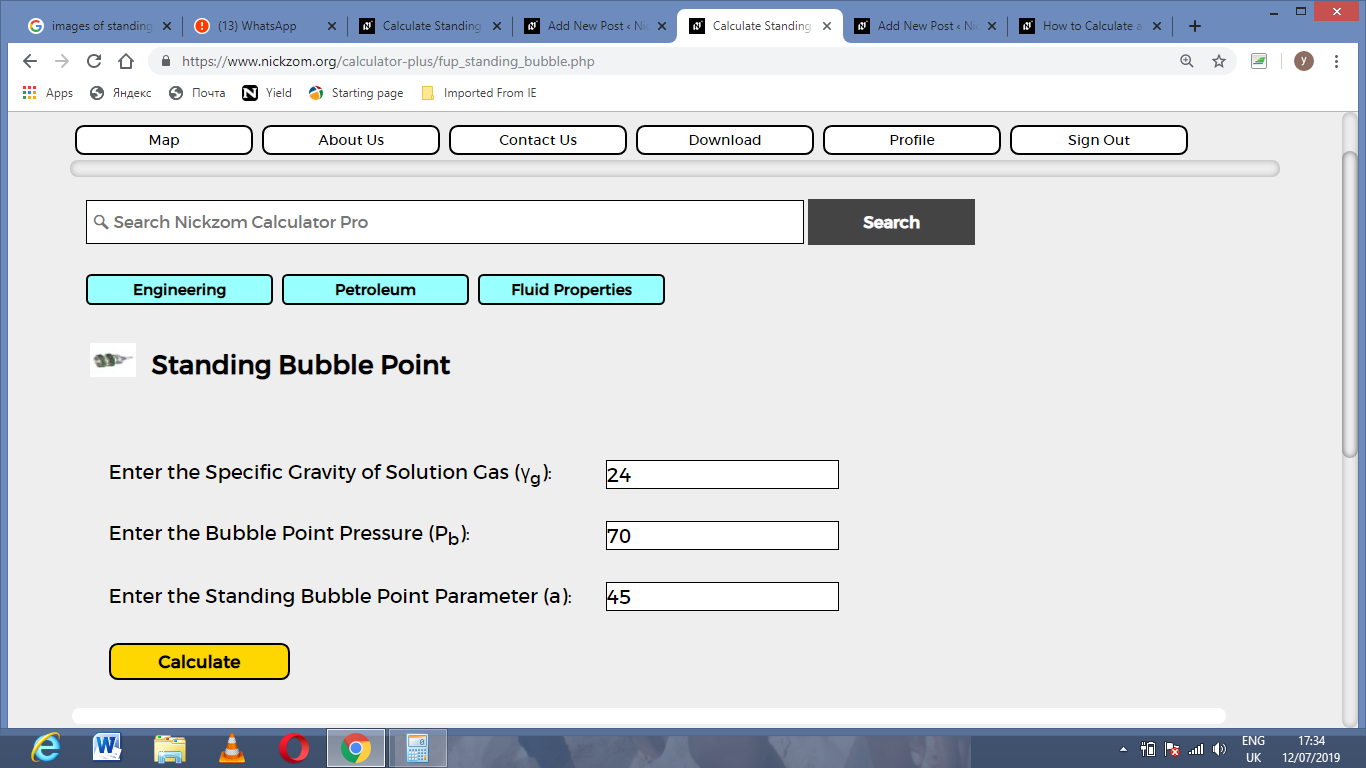Finally, Click on Calculate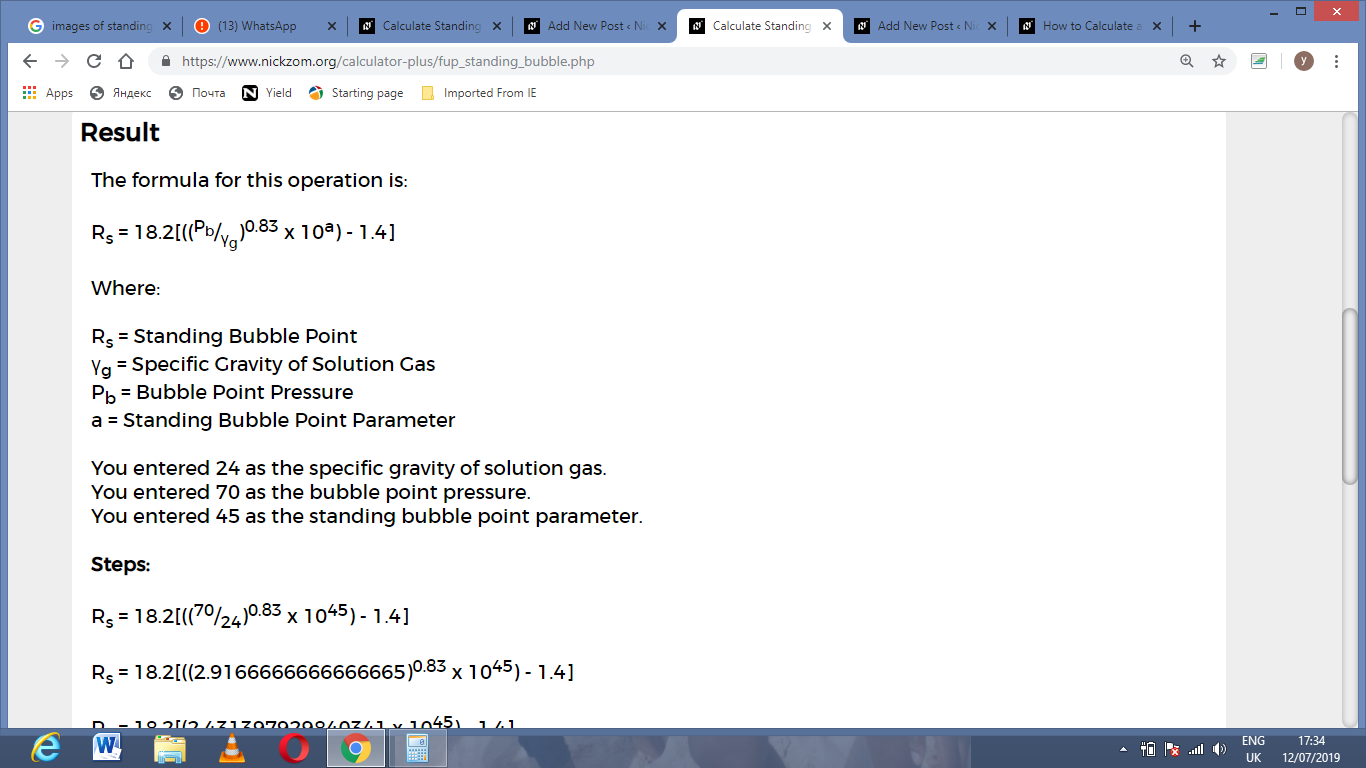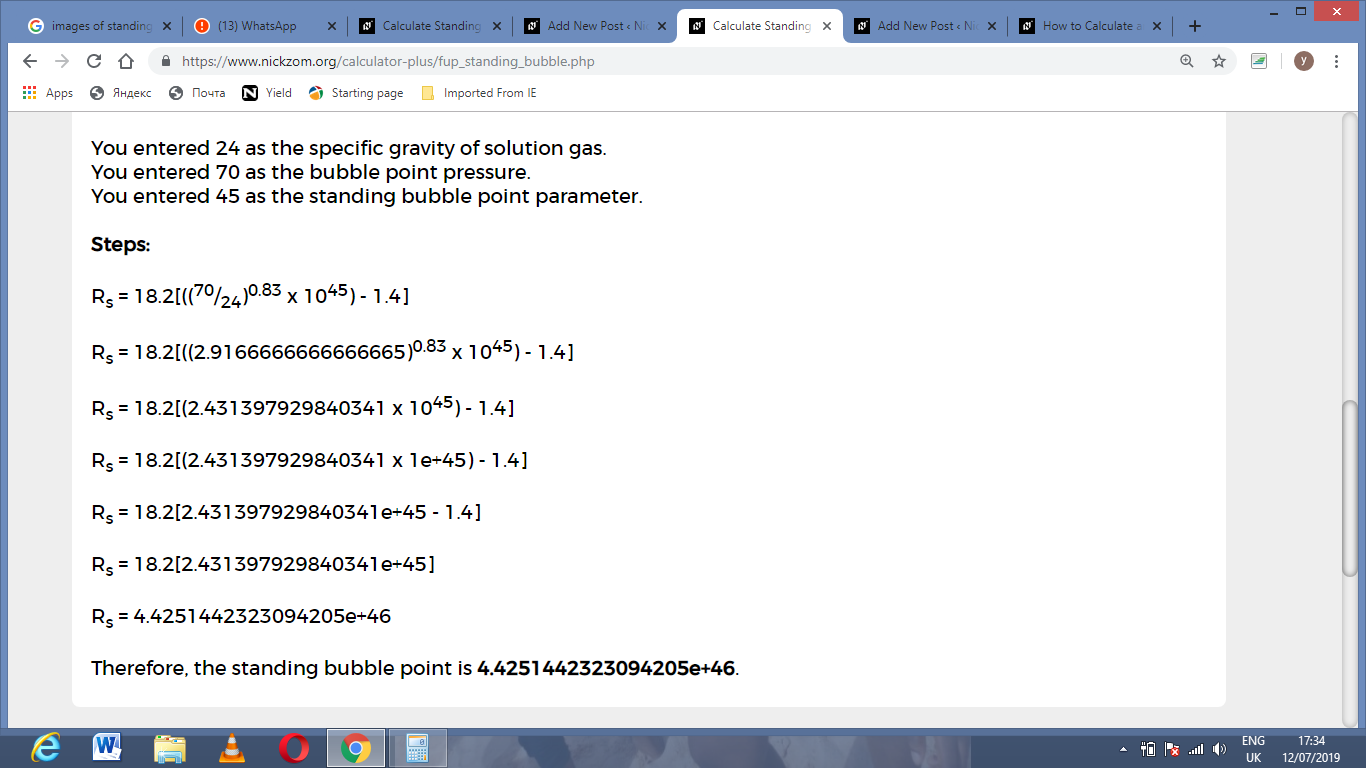As you can see from the screenshot above, Nickzom Calculator– The Calculator Encyclopedia solves for the standing bubble point and presents the formula, workings and steps too.